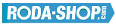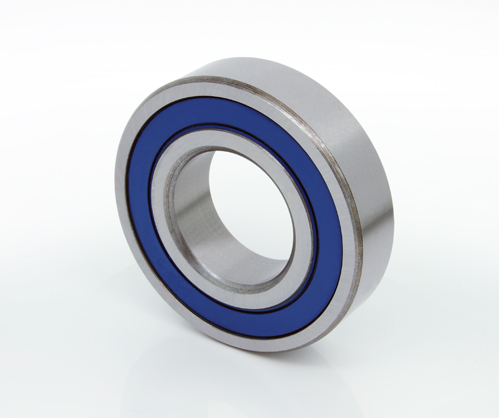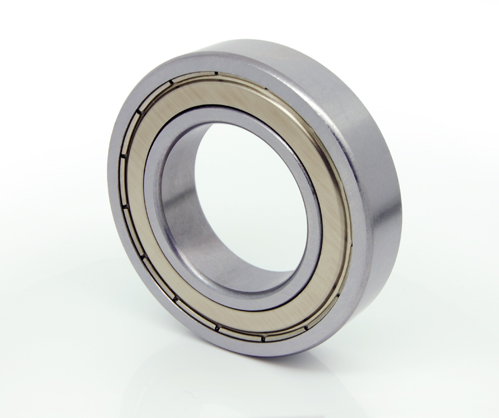# Chrome Steel Ball BearingsBall Bearing Chrome Steel 100Cr6(Nr.1.3505)

Manufacturer Product Image Item Name Price### 11211-TN

inside diameter =
outside diameter =
width =
€45.92### 60/22-2RS

60/22-2RS
inside diameter = 22
outside diameter = 44
width = 12
€8.50### 6202 2RS/16

inside diameter = 16
outside diameter = 35
width = 11
€7.74### 6408-2RS

inside diameter =
outside diameter =
width =
€12.25### 16001

Popular Ball Bearing Series Chrome Steel Open(No Shields) Dimension d=12.00 Dimension D=28.00 Dimension B=7.00 Dinamic Load Rating=4650 Static Load Rating=1980 Weight=0.022gr. Speed limit Grease=27.0 Speed limit Oil=0
inside diameter = 12.00
outside diameter = 28.00
width = 7.00
€4.54### 16001-2RS

Popular Ball Bearing Series Chrome Steel Rubber Seals on Both sides Dimension d=12.00 Dimension D=28.00 Dimension B=7.00 Dinamic Load Rating=4650 Static Load Rating=1980 Weight=0.022gr. Speed limit Grease=27.0 Speed limit Oil=0
inside diameter = 12.00
outside diameter = 28.00
width = 7.00
€4.54### 16001-2Z

Popular Ball Bearing Series Chrome Steel Metallic Shields on both sides Dimension d=12.00 Dimension D=28.00 Dimension B=7.00 Dinamic Load Rating=4650 Static Load Rating=1980 Weight=0.022gr. Speed limit Grease=27.0 Speed limit Oil=0
inside diameter = 12.00
outside diameter = 28.00
width = 7.00
€4.54### 16002

Popular Ball Bearing Series Chrome Steel Open(No Shields) Dimension d=15.00 Dimension D=32.00 Dimension B=8.00 Dinamic Load Rating=5590 Static Load Rating=2850 Weight=0.025gr. Speed limit Grease=22.0 Speed limit Oil=0
inside diameter = 15.00
outside diameter = 32.00
width = 8.00
€4.54### 16002-2RS

Popular Ball Bearing Series Chrome Steel Rubber Seals on Both sides Dimension d=15.00 Dimension D=32.00 Dimension B=8.00 Dinamic Load Rating=5590 Static Load Rating=2850 Weight=0.025gr. Speed limit Grease=22.0 Speed limit Oil=0
inside diameter = 15.00
outside diameter = 32.00
width = 8.00
€4.54### 16002-2Z

Popular Ball Bearing Series Chrome Steel Metallic Shields on both sides Dimension d=15.00 Dimension D=32.00 Dimension B=8.00 Dinamic Load Rating=5590 Static Load Rating=2850 Weight=0.025gr. Speed limit Grease=22.0 Speed limit Oil=0
inside diameter = 15.00
outside diameter = 32.00
width = 8.00
€4.54